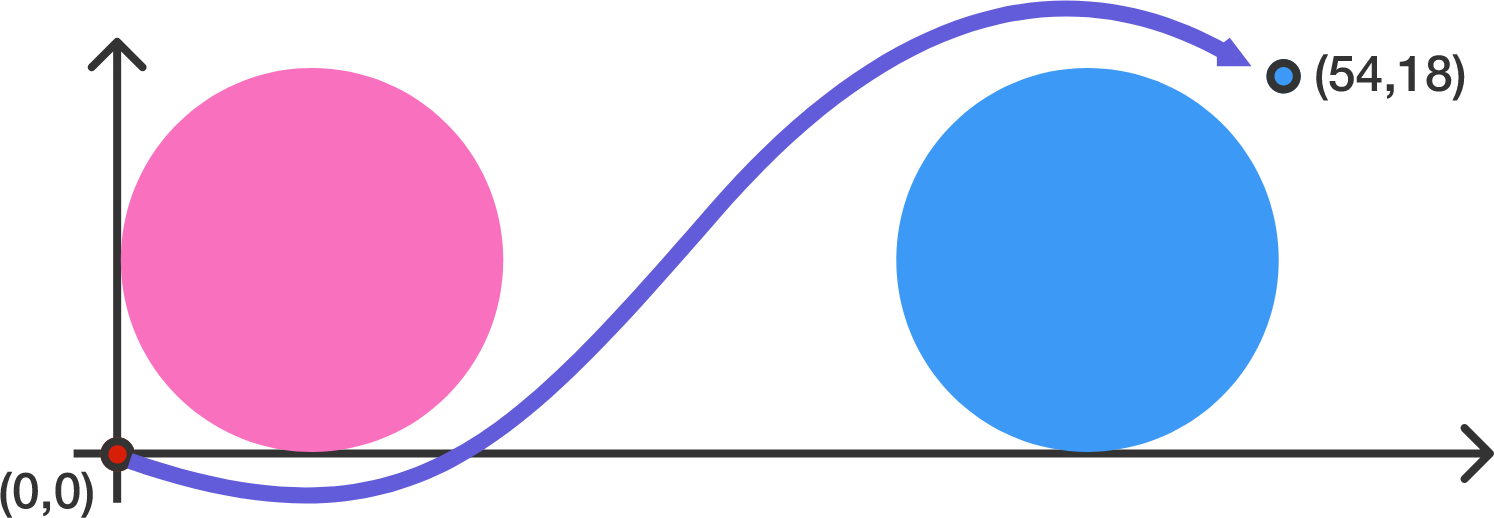# Avoid the Annoying Circular Objects

Geometry Level 2You are currently located on point $(0,0)$ and you want to get on point $(54,18)$. However, there are some annoying circular objects in the way! They are defined by $x^2+y^2-18x-18y+81=0$ $x^2+y^2-90x-18y+2025=0$

If you cannot walk through these annoying circular objects, then the shortest possible path possible to point $(54,18)$ can be expressed as $a+b\sqrt{c}+d\pi$ for positive integers $a,b,c,d$ with $c$ square-free. What is $a+b+c+d$?

×